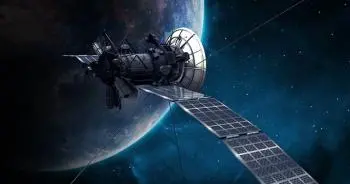# 10 Examples of Newton's First Law: the Law of InertiaNewton's first law, also known as the law of inertia, states that an object at rest will remain at rest and a moving object will continue to move at a constant speed in a straight line unless acted upon by a net external force

Here are some examples of Newton's first law:

## 1. Object at Rest

Imagine a book on a table. If no force is applied to the book, it will remain at rest on the table. According to Newton's first law, the book will remain at rest until an external force acts on it.

## 2. Moving Object

Suppose you push a grocery cart down an aisle and then release it. The cart will continue to move down the aisle for a while before gradually coming to a stop due to friction. This is because, once in motion, the cart tends to maintain its constant speed due to inertia.

In this case, the force of friction generates an acceleration in the opposite direction to that of the movement until it stops.

## 3. Space Flight

In space, where there are no significant forces to slow down a moving object, a spacecraft launched at a given speed will continue to move at that speed in a straight line.

Due to the law of universal gravitation, the force of gravity decreases with increasing distance between the aircraft and the Earth until a time comes when the gravitational force is insufficient to reduce the speed to zero. Therefore, it is considered that at a certain height, a spacecraft no longer experiences external forces that change its state of motion.

In the case of a satellite orbiting the Earth, a similar effect occurs, but in this case the gravitational force acts as a centripetal force, modifying the trajectory of the satellite. For this reason they have circular trajectories.

## 4. Parachute

When a person parachutes out of an airplane, they initially fall downward due to gravity. However, once the parachute deploys, it experiences air resistance that acts in the opposite direction of movement.

This causes a net external force upward and slows the rate of fall, allowing for a slower descent.

## 5. Ice Skating

If a skater slides on an ice rink without applying any additional force, he will continue to slide in a straight line at a constant speed. This is due to the lack of significant friction on the ice, allowing the skater to maintain their state of motion.

## 6. Rolling Ball

Imagine a ball rolling on a flat surface. If there are no external forces acting on the ball, it will continue to roll at a constant speed in a straight line. The inertia of the moving object maintains its movement without the need to apply more force.

## 7. Passengers in a Car

When you are in a moving car and it stops suddenly, you feel your body lean forward. This is because, according to Newton's first law, your body tends to maintain its state of motion and continues to move forward due to inertia, while the car comes to a stop.

## 8. Throwing a Projectile

When you throw an object, such as a ball, with a certain speed and angle, it will follow a ballistic trajectory. Once airborne, the projectile continues to move at a constant speed in a straight line until gravity and air resistance act on it.

As it happens in a parabolic shooting movement, the horizontal velocity remains constant because there is no external force that modifies it. In contrast, the vertical speed is affected by the force of gravity.

## 9. Spin of a Top

When you spin a top, it tends to maintain its axis of rotation and spin speed due to inertia. It will only stop or change its motion if an external force, such as friction or a blow, acts on it.

## 10. Planets in Orbit

The planets in our solar system follow elliptical orbits around the Sun due to the Sun's gravitational influence. According to Newton's first law, the planets continue to move in their orbits at constant speeds due to their inertia.

However, the reason why they describe an elliptical trajectory is the influence of an external force, the gravitational force of the Sun, which acts as a centripetal force.

Author:

Published: June 28, 2023
Last review: June 28, 2023